These Pages include various standards. To confirm the status of any standard, identify the replacement standard if it is obsolete and/or purchase the standard please use. BSI Shop It is also possible to become a BSI member and obtain copies of the Standards at much reduced prices.

Reinforced concrete beams subject to bending moments

The building structures pages have been added over the six months to Dec. 2012.     They are very much work-in-progress and I will be updating them on a regular basis over the next six months.

Introduction

This page includes note covering the theory of reinforced concrete beams.     The theory generally used in the past has bee based on elastic method of analysis.    In the last twenty years the elastic theory has beean superseded by the ultimate load method in the codes applicable to the construction industry.    The notes below consider , in outline, both methods.

symbols
 A s = Area of tension reinforcement A' s = Area of Compression reinforcement b = width or effective width of section b w = average width of web d = effective depth of section (compression face to centre of reinforcement ) x= depth to neutral axis of section Ic = Second moment of Area of transformed section C = resultant compressive force in concrete T = resultant tensile force in reinforcement d' = depth to compression reinforcement Ec = Youngs modulus for reinforcement Es = Youngs modulus for concrete nsc = modular ratio = Es /Ec h f = thickness of flange L = effective span of beam M = Design moment at section σc = Stress in concrete σs = Stress in reinforcement Mu = Design Ultimate moment at section Mrc = Moment of Resistance of beam based on concrete strength Mrs = Moment of Resistance of beam based on reinforcement strength

Elastic theory

The basis of this theory is provided on webpage Composite beams

Consider a rectangular beam subject to bending forces such that here are compressive forces on the top surface and tensile force on the bottom surface.     The beam is subject to a bending moment M and is reinforced in its tensile region by a number or reinforcement bars of total cross section are As. The centre of the reinfocement is at a depth d from the upper surface and is identified as the effective depth.    The neutral axis of the section is at a depth x from the upper surface.    The position of the neutral axis is to be calculated.    The concrete is assumed to only contribute to the strengh resisting bending in the compressive region above the neutral axis i.e all tensile forces are resisted by the steel reinforcement.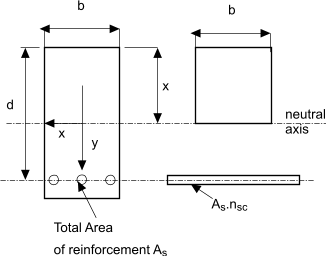The modular ratio nsc = Es /Ec. Multiplying the area of the reinforcement bars by the modular ratio results in the area reinforcement being effectively transformed into an equivalent area of concrete....Taking moments of areas about the neutral axis results in the equation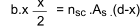This when rearranged results in the quadratic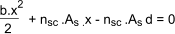The only relevant solution to this equation is the positive value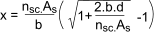The second moment of area Ic of the transformed section is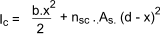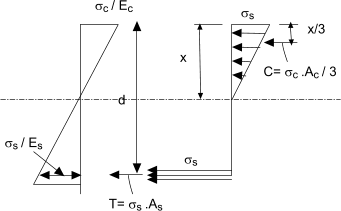The maximum stress in the concrete at the extreme fibre is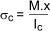The strain diagram shown above may be used to calculate the stress in the reinforcement.    The strain diagram is linear throughout the depth since the beam section is assumed to remain plane during bending. Therfore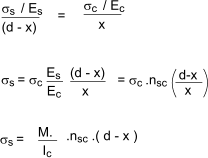Moment of Resistance of beam

This is the moment withstood by the beam when the stress in the concrete or the steel reaches the maximum allowable value.    The value for the concrete and steel is calculated easily from the stress diagram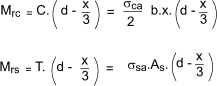The moment of resistance of the beam according to elastic theory is the lesser of Mrc or Mrs

The critical /economic section of a beam is when the reinforcement area is adjusted such that Mrs is equal to Mrc

Reinforcement in compression zone of beam

For a beam of given cross section dimensions there is a point when increasing the area of the reinforcement does not provide a resulting increase in the moment of resistance because the concrete becomes the limiting factor.    This limitation can be overcome by adding reinforcement in the compression zone of the beam.     Consider a beam as shown below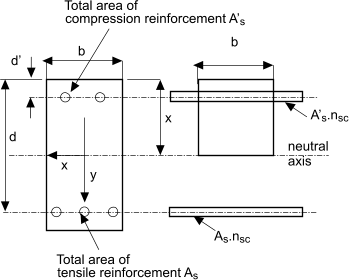Taking moments of area about the neutral axis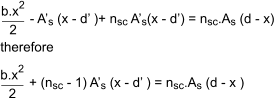The second moment of area of the transformed section is therefore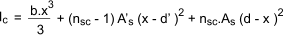The maximum stress in the concrete at the extreme fibre isThe stress in the tensile reinforcement and in the compressive reinforcement is calculated as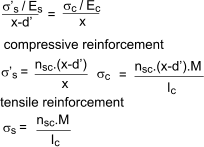Ultimate load theory for concrete beams is based on the calculated moment of resistance (ultimate moment of reistance) being based on the failure strength of concrete in compression and the yield strength of the reinforcement of the steel in tension both modified by a margin of safety.     However concrete generally fails suddenly while the failure of steel is generally progressive .    The margins are typically 1,5 for concrete and 1,15 for steel.    When the characteristic stength for concrete is based on the cube strength it is necessry that it is multiplied by 2/3 because the stength in bending is always significantly less than that resulting from the cube strength.

The design strength for concrete and steel in bending is therefore typically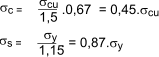The ultimate load theory analysis is based on the assumptions that plane surfaces remain plane during bending and there is no contribution to the bending strength of the beam from concrete in tension.    The strain therefore varies uniformly down the beam section.    The stress diagram however is not linear but has a rectangular parabolic shape as shown below.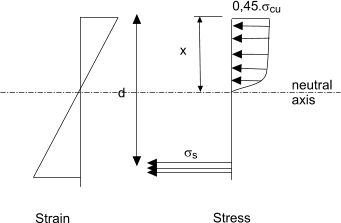A reasonably accurate approximation of this curve which is used in strength calculations is shown below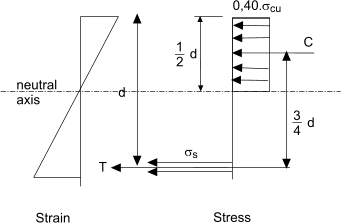The moment of resistance in this instance is identified as the ultimate moment of resitance Mu and is easily calculated as shown below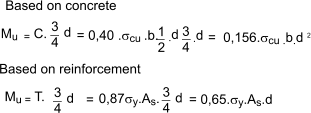The section considered above identifies the ultimate moment of resistance when the neutral axis is 0,5.d .  A lower design moment would have a requirement for a section with a reduced value of x to the neutral axis.     It is easily possible by evaluating moment of resistance of a section resulting from different values of the ratio of x/d by taking moments about the tensile reinforcement.
Note:The stress distribution, as used in the relevant code and as used for this exercise, is actually slightly different from that shown above.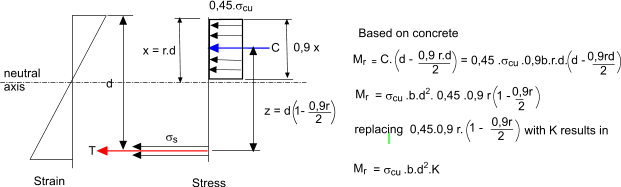A plot of K vs r= x/d is easily plottted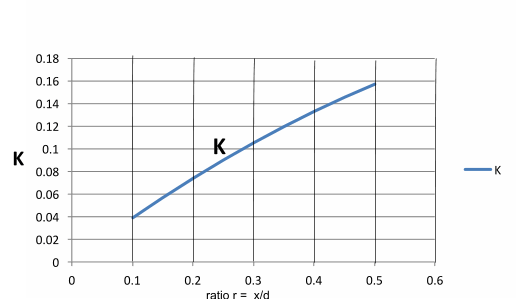r 0.13 0.15 0.2 0.25 0.3 0.35 0.4 0.45 0.5 K 0.04957 0.0566494 0.07371 0.0898594 0.105097 0.119424 0.13284 0.145344 0.156938 z 0.9415 0.9325 0.91 0.8875 0.865 0.8425 0.82 0.7975 0.775
 Useful relevant Links Mechanical programs....Includes Framework download - an advanced struct. analysis program Concrete beam strength -Strucsoft.... An applet used for determining strength of reinforced concrete beams C Capriani.. reinforced concrete beams... Extremely clear notes with worked examples C Capriani.. concrete flat flabs... Extremely clear notes with worked examples C Capriani..reinforced concrete columns... Extremely clear notes with worked examples Design of slabs beams and foundations using SAFE...Background theory for a software package ( including many codes) Design of centric loaded column...Detailed explanation including reference to Eurocode xcalcs... Section information and Calculations Free CD offer from reinforced Concrete Council... A comprehensive set of caluclators on CD to BS 8110 Reinforcement bar bending - kbrebar... Detailed dimensions for bar bending to BS8666